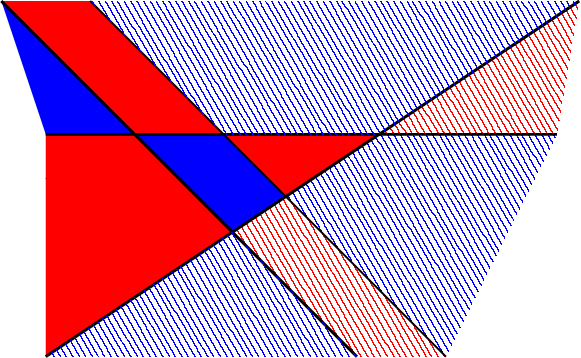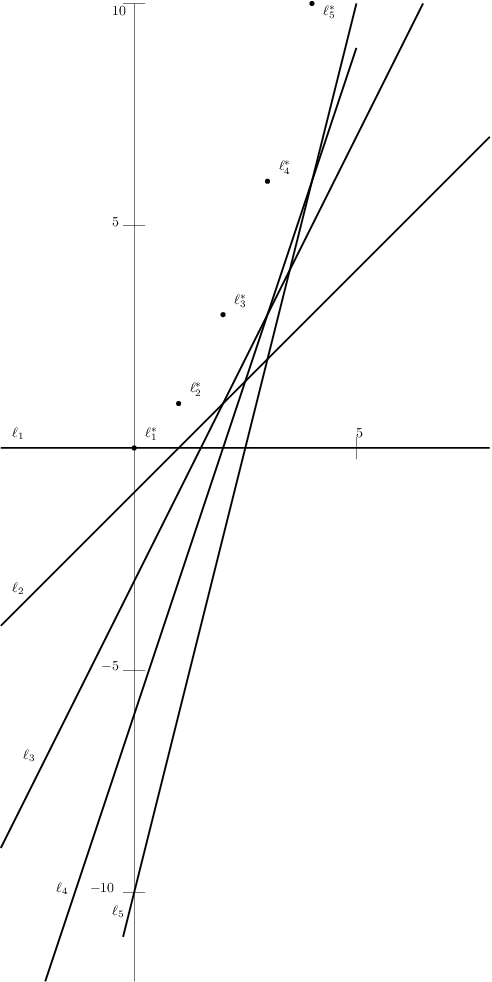# Internet Problem Solving Contest

## Solution to Problem J – Judicious cuts

The easy subproblem is really really easy. To the point that you’ll probably bang your head against the wall if you didn’t get it. The easiest solution: output r − 1 distinct horizontal lines.

We will now take a look at the hard subproblem.

First let’s look at how to count the number of regions for a given arrangement of lines. Our output format already ensures that there are no lines parallel to the y-axis, but in general, one can slightly rotate the plane to avoid those anyway. Consider any finite amount of non-vertical lines. We can now find the rightmost point of every region. There are two types of regions:

• Those that do not have a rightmost point, as they are unbounded on the right side. Since there are no lines parallel to the y-axis, it is easy to observe that there are exactly ℓ + 1 of these.
• Those that do have a rightmost point. Such rightmost point always lies at the intersection of two or more lines. We call these regions bounded.

In the figure below, the regions filled with stripes do not have a rightmost point – those are the unbounded regions. The other five filled with solid colour are bounded.When the lines are in a general position (that is, no three lines meet at a point and no two lines are parallel) then every pair of lines has a unique intersection point. Each intersection point serves as a rightmost point to exactly one region. Since there are ${\ell} \choose 2$ such intersections, the total number of regions is
$$\frac{\ell^2 + \ell + 2}{2}.$$
This is also the maximal amount of regions one can make using lines. How do parallel lines and multiple lines meeting at a point affect the result?

The case of parallel lines is simple – if there is a set of k parallel lines, we have $k\choose 2$ fewer intersection points which exactly corresponds to the number of regions we lose.

Let’s look at m lines meeting at a point and ignore all the others for a while. If the lines did not meet at a single point, but they were in general position instead, there would be $m \choose 2$ regions with a rightmost point. Now we have 1 intersection point that borders 2m regions. We already know that m + 1 of them are unbounded, which leaves us with m − 1 bounded regions. This means that the effect of an arrangement of m lines meeting in a single point on the number of regions is a decrease by ${m \choose 2} - (m - 1) = {m-1 \choose 2}$.

The above observations can now be used to count the number of regions for any configuration of lines. (This is sometimes called Roberts’ formula.)

This gives us an idea how to construct the solution for a given r. If there is an such that (ℓ2 + ℓ + 2)/2 = r, output lines in a general position. Otherwise, find the smallest for which the general position has more regions and try to remove some of them by introducing the “flaws” mentioned above. For example, if r = 13, we can take ℓ = 5 lines. If they were in a general position, we would have 16 regions. If we instead place them so that four of them meet at the same point, this will reduce the number of regions by ${4-1 \choose 2} = 3$, leaving us with 13 regions.

There are many different constructions, for instance one can introduce some sets of parallel lines, and ensure that they do not create points where three or more lines intersect. The solution presented below uses the notion of a point-line duality and is able to stay well within the limits on line parameters.

Formally a dual point to the line L given by y = mx + b is the point L* = (m, −b). Every line not parallel to y-axis has a dual point, and every point has a corresponding dual line. Duality has many interesting properties that are often useful in similar constructions. We use two of them here without proof:

• Two lines L1 and L2 are parallel iff their duals L1* and L2* have the same x coordinate.
• Three lines L1, L2, L3 meet at a single point iff their dual points L1*, L2*, L3* are collinear. The intersection point is the primal of the line connecting the dual points.

We can describe the construction and also prove its properties easier in the dual representation. In our construction we avoid parallel lines completely by setting mi (the slope of the i-th line) equal to i. We let the y-intercept increase by i between consecutive points. E.g., for five dual points we have {(0, 0),(1, 1),(2, 3),(3, 6),(4, 10)} (depicted in the following figure). What this construction effectively does is increasing the slope of the dual line between x consecutive dual points. It is easy to prove that no three such dual points lie on a dual line: since the slopes of lines connecting different pairs of dual points are different, so is the x-coordinate of intersection of their primals. When the intersection points have different x-coordinate, they are different points. Hence, no three (primal) lines meet at a (primal) point and this approach gives us lines in general position.How to decrease the number of regions? Let’s start by always outputting a primal line (0, 0). Let x denote the number by which we need to decrease the number of regions to achieve the desired amount. Once we reduce x to zero, we are done. In order to do that, we pick the largest c such that ${c-1 \choose 2} \leq x$. Introducing c collinear dual points reduces x to $x' = x - {c-1 \choose 2}$. The simplest and efficient way to introduce c collinear points is to add c − 1 points collinear with the last. We do this by keeping the same slope for all. For example, the last inserted point is (4, 4) and we demand 3 consecutive points. We use slope 5 for both, that is we output points/lines (5, 9), (6, 14). The point (6, 14) is now the last point, and we can use it for another set of collinear points (e.g., (7, 21), (8, 28)).

The thing left to do is to assess whether this construction is efficient enough. As introducing k collinear dual points removes Θ(k2) regions, and adding an extra line gives us Θ(ℓ) new regions, the intuition is that for large number of lines this construction is sufficient. A bit of case-work reveals the construction does not work for r ∈ {3, 5, 9, 12, 17, 24}, where one would aim to use (2, 3, 4, 5, 6, 7) lines, respectively.

In all cases but r = 5 and r = 17 one can fix the construction by using one set of parallel lines at the end (k parallel lines are sufficient to reduce r by $k \choose 2$, whereas k + 1 intersecting lines are needed; and we can again reuse the last inserted point1), or by hand-crafting the solutions. Case r = 17 can indeed be done using 6 lines, but even the more efficient construction fails to find the solution, we’re left with solution by hand.2

Finally, we’re left with r = 5. This is indeed a special case where we need one more line than the lower bound we established before. We’re left with no choice – the approach used for the easy subproblem is optimal here.

In the scope of the contest, it is advisable to leave the proof out, follow the intuition and then solve by hand the few cases where the construction failed. If we’re lazy, we can drop the negative sign by y-intercept – this corresponds to reflection of the dual along x-axis, which definitely doesn’t affect the solution and the properties of the duality we’re using.

Bonus question: What if parallel lines were not allowed?

1. Notice that when using just parallel lines, one cannot reuse the last point for the next set, as parallelity is transitive. It is also harder to prevent intersecting lines.

2. Hint: one set of 2 parallel lines, one set of 3 parallel lines, and a 3-way intersection.